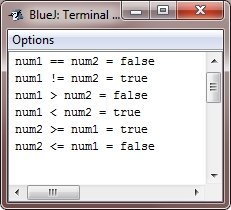# Java Relational Operators

The relational operators determine the relationship that one operand has to the other.

Specifically, they determine equally and ordering. Here, the following table lists the relational operators available in Java:

Operator Name Meaning
== Equal to This operator is used to check whether the value of the two operands are equal or not. If yes then the condition becomes true, otherwise false
!= Not equal to This operator is used to check whether the value of the two operands are equal or not. If the values are not equal, the condition becomes true, otherwise false
> Greater than This operator is used to check whether the left operand's value is greater than the right operand's value or not. If yes, then the condition becomes true, otherwise false
< Less than This operator is used to check whether the left operand's value is less than the right operand's value or not. If yes, then the condition becomes true, otherwise false
>= Greater than or equal to This operator is used to check whether the left operand's value is greater than or equal, to the right operand's value or not. If yes, then the condition becomes true, otherwise false
<= Less than or equal to This operator is used to check whether the left operand's value is less than or equal, to the right operand's value or not. If yes, then then condition becomes true, otherwise false

The outcome of these operations is a boolean value. The relational operators are most frequently used in the expressions that control the if statement and the various loop statements.

Any type in Java, including integers, character, floating-point numbers, and Booleans can be compared using the equality test(==), and the inequality test (!=). Notice that in Java equality is denoted with two equal signs, not once.

Remember - A single equal sign is the assignment operator.

Only numeric types can be compared using the ordering operators. That is, only integers, floating-point, and character operands may be compared to see which is greater or less than the other.

As stated, the result produced by a relational operator is a boolean value. For example, the following code fragment is perfectly valid:

```int a = 4;
int b = 1;
boolean c = a < b;```

In this case, the result of a<b (which is false) is stored in c.

If you are coming from C/C++ background, please not the following. In C/C++, these types of statements are very common :

```int done;
// ...
if(!done)...  // Valid in C/C++
if(done)...   // but not in Java.```

In Java, these statements must be written like this :

```if(done == 0)...   // This is a Java-style.
if(done != 0)...```

The reason is that Java does not define true and false in the same way as C/C++. In C/C++, true is any nonzero value and false is zero. In Java, true and false are non-numeric values that do not relate to zero or nonzero. Therefore, to test for zero or nonzero, you must explicitly employ one or more of the relational operators.

## Relational Operators Example

The following example program demonstrates the relational operators:

```/* Java Program Example - Java Relational Operators */

public class JavaProgram
{
public static void main(String args[])
{

int num1 = 100, num2 = 200;

System.out.println("num1 == num2 = " + (num1 == num2) );
System.out.println("num1 != num2 = " + (num1 != num2) );
System.out.println("num1 > num2 = " + (num1 > num2) );
System.out.println("num1 < num2 = " + (num1 < num2) );
System.out.println("num2 >= num1 = " + (num2 >= num1) );
System.out.println("num2 <= num1 = " + (num2 <= num1) );

}
}```

When the above Java program is compile and executed, it will produce the following output:Java Online Test

« Previous Tutorial Next Tutorial »